www.batmath.it

### A more formal definition

It turns out that, in order to give a formal definition of limit, it's better to begin investigation from the codomain instead of the domain.

More precisely, given an accumulation point c for the domain D of a function f, we said that the limit is the answer to the following question: what is the behaviour of f when x approaches c? Here the focus is on the variable x that "moves" towards c. In order to formalize this definition it turns out that we are forced to focus on the possible values of the function: given a number l, try to find the set A of all elements x of the domain whose images are close to l. If all the points sufficiently close to c belong to A, then l is the limit, otherwise it is not.

This requires a precise definition of what we mean by "close". For the purpose of our simplified theory of limits, this definition can be concentrated as follows:

• Given a real number c, we call neighbourhood of c an open interval containing c.
• An interval like ]c,+∞[ is a neighbourhood of +∞.
• An interval like ]-∞,c[ is a neighbourhood of -∞.

So, given a real number c and one of its neighbourhoods, say ]a,b[, every number belonging to ]a,b[ is close to c, other numbers are not close to c. The neighbourhood is a measure of "closeness": changing the neighbourhood affects the property of being close or not to c.

We can now give the formal definition of limit:Given a function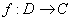, and an accumulation point c for D (+∞ and -∞ are allowed), we say that l (+∞ and -∞ are allowed also for l) is the limit of the function as x tends to c, if for every neighbourhood U of l we are able to find a neighbourhood V of c such that the values of the function f for all points xV, c excepted, belong to U.

In the following picture you can see a graphical representation of what happens when both c and l are numbers, that is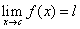.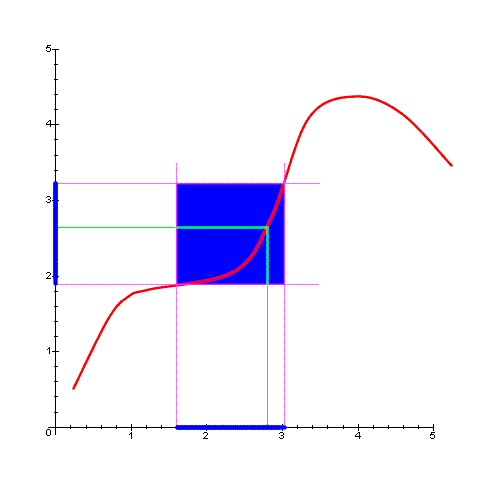In the folllowing picture you can see a graphical representation of what happens when c is a number and l is +∞, that is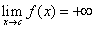.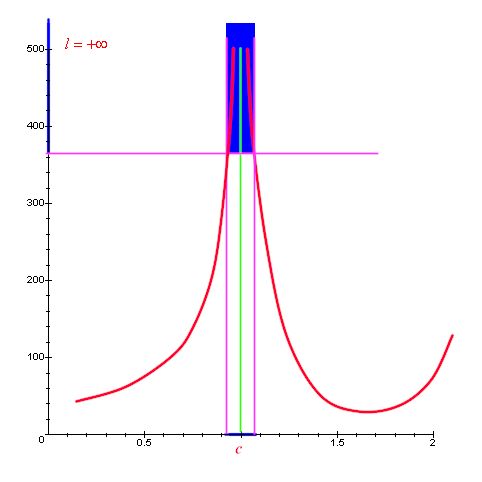In the following picture you can see a graphical representation of what happens when both c and l are +∞, that is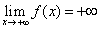.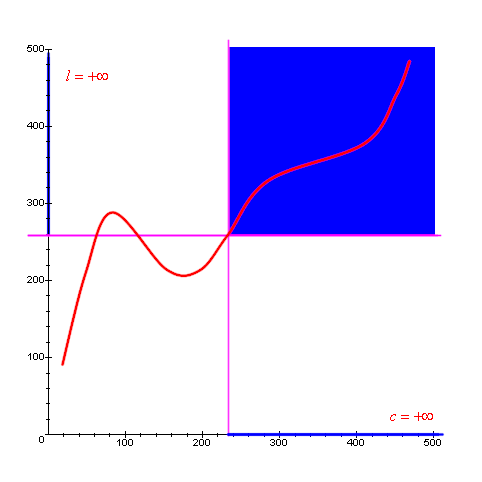first published on march 26 2002 - last updated on september 01 2003